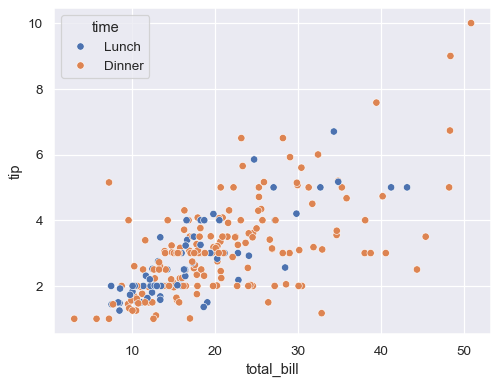# Is it possible to switch the position of the labels and handels(artists) inside a legend of a matplotlib / pyplot / seaborn plot?

The following code creates a plot with a legend (example taken from https://seaborn.pydata.org/generated/seaborn.scatterplot.html)

```import seaborn as sns
tips = sns.load_dataset("tips")
sns.scatterplot(data=tips, x="total_bill", y="tip", hue="time")
```Is it possible to switch the position of the handles(artists) and labels INSIDE the legend?

The artists are the blue and the orange dot and the labels is “Lunch” and “Dinner”. I would like to have “Lunch” and “Dinner” on the left inside the legend and the colored dots on the right inside the legend.

## Answer

For the scatterplot, you can create the legend again with `markerfirst=False`:

```import seaborn as sns
from matplotlib import pyplot as plt

sns.set()
tips = sns.load_dataset("tips")
ax = sns.scatterplot(data=tips, x="total_bill", y="tip", hue="time")
ax.legend(markerfirst=False, title='Time')
plt.show()
```